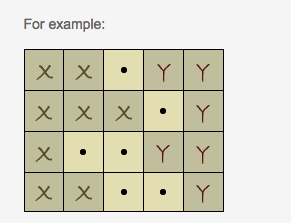# Details(CODEEVAL)

## CHALLENGE DESCRIPTION:

There are two details on a M*N checkered field. The detail X covers several (at least one first cell) cells in each line. The detail Y covers several (at least one last cell) cells. Each cell is either fully covered with a detail or not.The detail Y starts moving left (without any turn) until it bumps into the X detail at least with one cell. Determine by how many cells the detail Y will be moved.

## INPUT SAMPLE:

```XX.YY,XXX.Y,X..YY,XX..Y
XXX.YYYY,X...Y..Y,XX..YYYY,X.....YY,XX....YY
XX...YY,X....YY,XX..YYY,X..YYYY
XXYY,X..Y,XX.Y```

```1
1
2
0```

## CONSTRAINTS:

1. The matrices can be of different M*N sizes. (2 <= M <= 10, 2 <= N <= 10)
2. Number of test cases is 40.

## My Code

```#!/usr/bin/env ruby -w

def y_moving_size(lines)
min_size = lines.first.size
lines.each do |line|
x_index = line.rindex("X")
y_index = line.index("Y")
size = y_index - x_index - 1
min_size = size if size < min_size
return 0 if min_size == 0
end
min_size
end

ARGF.each_line do |line|
puts y_moving_size(line.chomp.split(","))
end
```